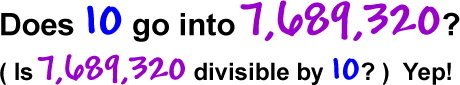Is 378 divisible by 2?

Is 729 divisible by 10?

Is 590 divisible by 5?

Is 83,497 divisible by 2?

Is 972,552 divisible by 5?

Is 290 divisible by 10?

The next two take a bit of math, but, once you get good at them, you can do them in your head!

Divisibility by 3 Test:

 A number is divisible by 3 if thesum of its digits is divisible by 3.

HUH?!

Don't worry -- this is easy!